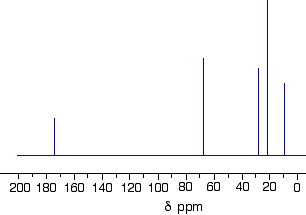# Carbon-13 NMR

## Key Questions

• The most useful information you can get from a $\text{^13"C}$ NMR spectrum is the number of non-equivalent carbon atoms.

For example, you have the $\text{^13"C}$ NMR spectrum of an unknown ester.Is its structure (a) CH₃CH₂COOCH₂CH₂CH₃, (b) CH₃CH₂COOCH(CH₃)₂, or (c) CH₃COOC(CH₃)₃?

You quickly calculate that

(a) has 6 different C atoms;
(b) has two equivalent C atoms, so there only 5 different carbon environments;
(c) has three equivalent C atoms, so there only 4 different carbon environments.

The spectrum shows 5 carbon signals, so the ester must be (c) isopropyl propanoate.

See below.

#### Explanation:

• $160 - 220 \textcolor{w h i t e}{X} \text{C=O}$
• $115 - 150 \textcolor{w h i t e}{X} \text{C=C, C≡N}$
• $10 - 90 \textcolor{w h i t e}{X X} \text{Alkane C}$

Each of these ranges is further subdivided. For example,

160-220 ppm Range

• $205 - 220 \textcolor{w h i t e}{X} \text{RCOR}$
• $190 - 200 \textcolor{w h i t e}{X} \text{RCHO}$
• $160 - 185 \textcolor{w h i t e}{X} \text{RCOOH, RCOOR}$

10-90 ppm Range

• $50 - 90 \textcolor{w h i t e}{X} \text{RCH"_2"O}$
• $30 - 60 \textcolor{w h i t e}{X} \text{RCH"_2"Cl}$
• $25 - 35 \textcolor{w h i t e}{X} \text{R"_3"CH}$
• $16 - 25 \textcolor{w h i t e}{X} \text{RCH"_2"R}$
• $10 - 15 \textcolor{w h i t e}{X} {\text{RCH}}_{3}$

These ranges are often presented in a chart like the one below.Even this limited information might enable you to match the carbon atoms to their corresponding peaks in the NMR spectrum of methyl methacrylate.For a start, spin active $C$ nuclei are much less common than spin active proton nuclei (the standard isotope is near 100% abundant). Given the prevalence of spin active protons, connectivity of the molecule may be determined.

#### Explanation:

It is a fundamental, underlying principle of all spectroscopy that the more absorbing species that exist in a molecule, the greater the observed signal. The standard proton is almost 100% abundant, whereas the spin active carbon nucleus is approx. 1% abundant (the remaining 99% are non-spin active isotopes). To a first approximation proton NMR spectroscopy should be much more sensitive than carbon NMR spectroscopy, and indeed it is. A proton NMR spectrum can be acquired in about 5 minutes with good signal to noise; carbon NMR spectra typically take a couple of hours (or overnight) and the signal to noise is much poorer. The proton nucleus is intrinsically much more sensitive than this in NMR experiments.

Also, since protons couple to each other, that is the adjacent, chemically different protons influence the appearance of the protons you are observing, a great deal of information may be gleaned as to the connectivity of your compound. By contrast, spin active carbon nuclei are observed in isolation (because they are so much less abundant; mind you, attached protons do modify the signal predictably), and the adjacent carbon does not influence the signal of the carbon you are observing.

All this being said, carbon NMR spectroscopy is used as a tandem technique with proton NMR spectroscopy. An organic chemist who makes a new compound would report its solution spectrum in both the carbon and the proton domains, as well as other characterizing data (melting point, mass spectrum, combustion analysis). Without these details, claims of a new compound would not be accepted.